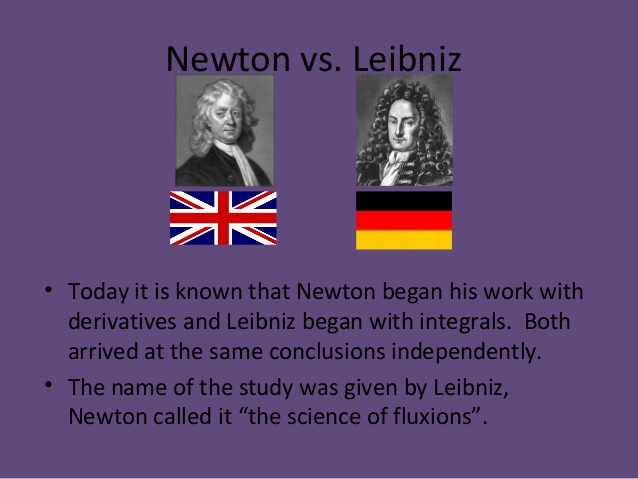## Newton’s Method

Newton’s method is one of the foundational concepts in numerical mathematics; it is a method for finding the minimum value of real-valued functions through a series of successive approximations.  While the approach has limitations (it can be “trapped” in local minimum, it can be slow, …) it is the gateway algorithm(1).

## Newton and Model-Based Design

Aside from the equations of motion, integration, theory of gravity (to start a long list) the children of Newton’s method are found in optimization problems. Optimization problems seek to find the minimize (or maximize) a value for a set of equations (or input data) based on the system input. Let’s consider an example of my Chevy Volt and optimizing energy use during my daily commute to work.Apparently you to can have a “Newton’s apple tree”
• Distance to work
• Range of battery
• Effect of starting battery temperature
• Driving conditions (highway / surface streets)
• Cabin climate control (heating / cooling)
• Cost of
• Charging the vehicle (at home / at work)
• Gas (in the Volt the gas engine charges the battery)
• Externalities
• Carbon emissions from the different energy sources.

When I frame this equation I set the commute as a “From-work-to-home-from-home-to-work” route. This, and thank you MathWorks, because there are free charging stations at my office, therefore any charging there is “free” (2).

• cost = C1 + C2 + C3
• C1 = Cost_per_Kilowatt_Work * Charge + E1
• C1 is at work co Cost_per_KW = 0 + E1 * Charge
• C2 = Cost_per_Kilowatt_Home * Charge + E2
• C2 = (0.12 + E2) * Charge
• C3 = Cost_Per_Gallon * Gallons_Per_Kilowatt * Charge + E3
• C3 = (3.50 * 30 + E3) * Charge

In this case the E1, E2 and E3 are the external costs for use of power. I don’t directly pay these costs(3) but from an ethical standpoint let us remember them.

The total charge is a function of distance, driving conditions and environmental conditions (do I run the heat or AC).

• Charge = F(Distance,DriveCond) + F(Cabin,Environment)

Skipping the analysis here to the conclusions. Since heating a car from the battery is very energy intensive, and since driving above 40 has a hit on fuel economy the “target” for short drives is to pre-heat the car (while at work) and to drive in a most sedate fashion. For medium drives the key is to use the engine heat the car and battery up at the start of the drive…

## The “costs” of our decisions:

In the equations above there are “E” terms assigned to each of the costs, the “externalities” in economic terms. These “E” terms can be used to “weigh” the optimization function to push (or pull) the outcome towards a given solution. In some cases the value of the weights can be calculated, other times they are assigned based on a desired outcome. For example, if I was creating an optimization equation for the “ultimate chocolate bar” I would have a heavy penalty against coconut; there is nothing inherently wrong about coconut I just can’t stand it.

## Integrating content in this post (4)

This post was written due to N simple observations

• Complex problems can be first understood using “base” methods: Real world optimization routines rarely used a Newton’s method due to efficiency issues, however for understanding optimization the simplicity of a Newton’s method can’t be beat.
• Concepts are Queen / King: The concept behind the Newton’s method, that successive approximations can lead to a numerical solution underpins multiple fields, optimization, feedback loops for controls, noise reduction…
• Reviewing “base” methods can lead to new understanding: In writing this post, and reviewing information on optimization and the basis of calculus (5) I have figured out solutions to a few problems that are plaguing me now, that of course will be in a future postI wish it was still called the “science of fluxions”

## Footnotes

1. The gateway numerical method: Next thing you know you will be diving into implicit and explicate solutions to P.D.E.s
2. There is still an external cost for charging at work, that energy is produce somewhere so some amount of greenhouse gas is being produced. This sort of “local” or self optimization can be seen as a leading cause of global climate change.
3. This isn’t completely true, since I breath I do pay a direct cost to my health due to air pollution.
4. What post featuring Newton would be complete without integration
5. Calculus: from Latin, literally ‘small pebble’, with enough “small pebbles” you can “rock” the mathematical world.

If you find value in these posts, consider subscribing

This site uses Akismet to reduce spam. Learn how your comment data is processed.